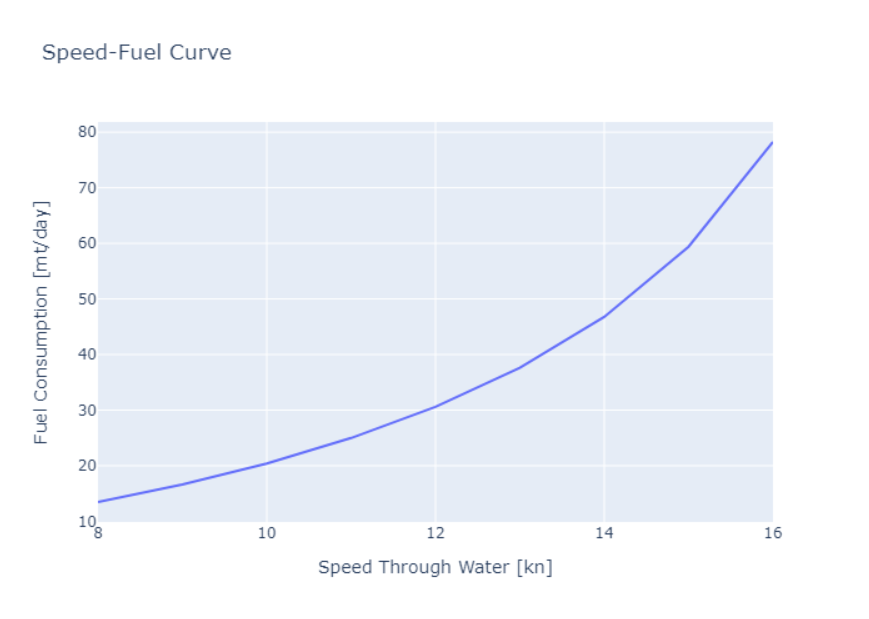# How to run this?

Go here to instantly run all code below inside of your browser.

# Use case

In the basics use case we demonstrated how to simulate a ship's performance in a single condition at a single speed.

We will extend this example and simulate a ship's performance over multiple speeds. This will allow us to construct a speed-fuel table and speed-fuel curve, showing the required fuel consumption for each speed of the ship.

## Authorize

Let's first make sure we're authorized to use the API. Fill in your API key and run the following code.

If everything is alright, you should see a list of ships.

``````API_KEY = "your-api-key"
``````
``````import json
import requests

API_URL = "https://api.toqua.ai"

url = "https://api.toqua.ai/ships/"
headers = {"accept": "application/json", "X-API-Key": API_KEY}

print(json.dumps(response.json(), indent=2))

``````
``````[
{
"name": "Trial Vessel",
"imo_number": 9999999,
"type": "Tanker",
"class": null,
"country": "SC",
"build_year": 2015,
"shipyard": "Toqua Shipyard",
"dwt": 220000.0,
"beam": 55.0,
"loa": 300.0,
"mcr": null,
"max_rpm": null,
"uuid": "eycrYbrzJNsJecGqKraUCn"
}
]
``````

Fill in the IMO number of the ship you want to analyze.

``````IMO_NUMBER = 9999999
``````

## Conditioning parameters

Let's again define our conditions.

``````wind_speed = 6          # [m/s]
wind_direction = 180    # [degrees]
wave_height = 2         # [m]
wave_direction = 90     # [degrees]
current_speed = 0.5     # [m/s]
current_direction = 0   # [degrees]
mean_draft = 20         # [m]
trim = -1               # [m]
fuel_specific_energy  = 41.5 # [MJ/kg]
``````

Our entrypoint will this time be a list, rather than a single value. We will analyze the ship's fuel consumption in speeds ranging from a STW of 8 knots to 16 knots.

``````stw = list(range(8, 17))

print(stw)
``````
``````[8, 9, 10, 11, 12, 13, 14, 15, 16]
``````

## Define the API input

Remember that the Toqua API expects the model input to look like this:

``````{
"date": "...",
"data": {
"stw": [...],
"draft_avg": [...],
"trim": [...],
"wave_direction": [...],
"wave_height": [...],
"wave_period": [...],
"current_speed": [...],
"current_direction": [...],
"wind_direction": [...],
"wind_speed": [...],
"fuel_specific_energy": [...]
}
}
``````

We will again ignore the `date` parameter for now.

Each parameter expects a list of values and all lists must have exactly the same length.
The parameter value at each index i corresponds to the parameter of each condition i.

Only the `stw` parameter is currently a list and we are only simulating a single condition, so we will have to duplicate the conditioning parameters once for each element in the `stw` list.

``````length_input = len(stw)

api_input = {
"data": {
"stw": stw,
"wave_direction": [wave_direction]*length_input,
"wave_height": [wave_height]*length_input,
"wind_direction": [wind_direction]*length_input,
"wind_speed": [wind_speed]*length_input,
"current_direction": [current_direction]*length_input,
"current_speed": [current_speed]*length_input,
"draft_avg": [mean_draft]*length_input,
"trim": [trim]*length_input,
"fuel_specific_energy": [fuel_specific_energy]*length_input
}
}
``````

## Query the API

``````def query_api(imo_number, payload):
url = f"https://api.toqua.ai/ships/{imo_number}/models/latest/predict"
"accept": "application/json",
"content-type": "application/json",
"X-API-Key": API_KEY,
}
return response

``````

Let's look at the values the model predicts.

``````response = query_api(IMO_NUMBER, api_input)

print(response.json())

``````
``````{'sog': [7.02808, 8.02808, 9.02808, 10.02808, 11.02808, 12.02808, 13.02808, 14.02808, 15.02808], 'stw': [8.0, 9.0, 10.0, 11.0, 12.0, 13.0, 14.0, 15.0, 16.0], 'me_rpm': [27.46916628911526, 30.06564265987471, 32.777084317488104, 35.616692448646226, 38.595463752209604, 41.722914386818964, 45.0076035987461, 48.457480299004324, 52.08011540213334], 'me_power': [2944.4400390625, 3692.91328125, 4625.341796875, 5781.925, 7210.34609375, 8966.95703125, 11118.1609375, 13741.9890625, 16929.89375], 'me_fo_consumption': [13.468422067490733, 16.596091186292796, 20.38510203019365, 24.97668192530601, 30.59028036155168, 37.60967357895273, 46.75443594824631, 59.40862324742315, 78.2326173179663], 'me_fo_emission': [42.701632164979365, 52.6179071061413, 64.63096598672897, 79.1885700441827, 96.9864838862996, 119.24147008206961, 148.2349391739149, 188.3550400059551, 248.03651320661214]}
``````

## Speed-Fuel Table

Using the Pandas library we can transform this output into table format to make it easier on the eyes, and for future data transformations.

``````import pandas as pd

df = pd.DataFrame(response.json())
df
``````
sog stw me_rpm me_power me_fo_consumption me_fo_emission
0 7.02808 8.0 27.469166 2944.440039 13.468422 42.701632
1 8.02808 9.0 30.065643 3692.913281 16.596091 52.617907
2 9.02808 10.0 32.777084 4625.341797 20.385102 64.630966
3 10.02808 11.0 35.616692 5781.925000 24.976682 79.188570
4 11.02808 12.0 38.595464 7210.346094 30.590280 96.986484
5 12.02808 13.0 41.722914 8966.957031 37.609674 119.241470
6 13.02808 14.0 45.007604 11118.160938 46.754436 148.234939
7 14.02808 15.0 48.457480 13741.989063 59.408623 188.355040
8 15.02808 16.0 52.080115 16929.893750 78.232617 248.036513

There we have our speed-fuel table. For speeds ranging from 8 to 16 knots it tells us the predicted fuel consumption in the conditions we defined earlier.

## Speed-Fuel Curve

Finally, to make things more tangible we can use the Plotly library to visualize our table as a speed-fuel curve.

``````import plotly.express as px

fig = px.line(df, x="stw", y="me_fo_consumption", title='Speed-Fuel Curve')
fig.update_layout(xaxis_title='Speed Through Water [kn]',
yaxis_title='Fuel Consumption [mt/day]')
fig.show()
``````